Test: Time Domain Analysis of Control Systems- 3

# Test: Time Domain Analysis of Control Systems- 3 - Electrical Engineering (EE)

Test Description

## 20 Questions MCQ Test GATE Electrical Engineering (EE) 2024 Mock Test Series - Test: Time Domain Analysis of Control Systems- 3

Test: Time Domain Analysis of Control Systems- 3 for Electrical Engineering (EE) 2023 is part of GATE Electrical Engineering (EE) 2024 Mock Test Series preparation. The Test: Time Domain Analysis of Control Systems- 3 questions and answers have been prepared according to the Electrical Engineering (EE) exam syllabus.The Test: Time Domain Analysis of Control Systems- 3 MCQs are made for Electrical Engineering (EE) 2023 Exam. Find important definitions, questions, notes, meanings, examples, exercises, MCQs and online tests for Test: Time Domain Analysis of Control Systems- 3 below.
Solutions of Test: Time Domain Analysis of Control Systems- 3 questions in English are available as part of our GATE Electrical Engineering (EE) 2024 Mock Test Series for Electrical Engineering (EE) & Test: Time Domain Analysis of Control Systems- 3 solutions in Hindi for GATE Electrical Engineering (EE) 2024 Mock Test Series course. Download more important topics, notes, lectures and mock test series for Electrical Engineering (EE) Exam by signing up for free. Attempt Test: Time Domain Analysis of Control Systems- 3 | 20 questions in 60 minutes | Mock test for Electrical Engineering (EE) preparation | Free important questions MCQ to study GATE Electrical Engineering (EE) 2024 Mock Test Series for Electrical Engineering (EE) Exam | Download free PDF with solutions
 1 Crore+ students have signed up on EduRev. Have you?
Test: Time Domain Analysis of Control Systems- 3 - Question 1

### For the given series RLC circuit, damping ratio (ξ) and undamped natural frequency (ωn) are respectively given by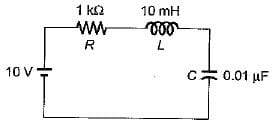Detailed Solution for Test: Time Domain Analysis of Control Systems- 3 - Question 1

The characteristic equation of series RLC circuit is given by: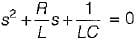Comparing with s2 + 2ξωns + ωn2 = 0, we have: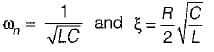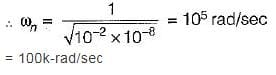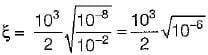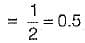Test: Time Domain Analysis of Control Systems- 3 - Question 2

### For the unity feedback control system shown below, by what factor the amplifier gain K should be multiplied so that the damping ratio is increased from 0.3 to 0.9?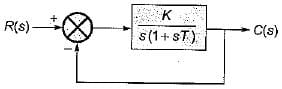Detailed Solution for Test: Time Domain Analysis of Control Systems- 3 - Question 2

Characteristic equation of given system is
1 + G(s) H(s) = 0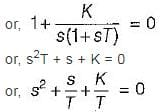Here,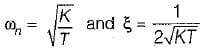For ξ1 = 0.3, gain = K1
For ξ2 = 0.9, gain = K2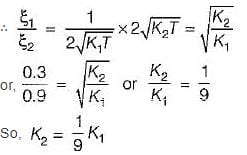Test: Time Domain Analysis of Control Systems- 3 - Question 3

### A system is shown in figure below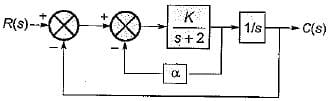What are the values of K and α respectively so that the system has a damping ratio of 0.7 and an undamped natural frequency ωn of 4 rad/sec ?

Detailed Solution for Test: Time Domain Analysis of Control Systems- 3 - Question 3

From given block diagram, the closed loop transfer function is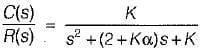Characteristic equation is,
s2 + (2 + Kα) s + K = 0
Here, ωn = -√K
and 2ξωn = (2 + kα)
∴ K = 16
Also, ξ = 0.7
∴ 2 x 0.7 x 4 = (2 + 16α)
or, (2 + 16α) = 5.6
or,
α = 3.6/16 = 0.225

Test: Time Domain Analysis of Control Systems- 3 - Question 4

For type-2 system, the steady state error due to parabolic input is

Detailed Solution for Test: Time Domain Analysis of Control Systems- 3 - Question 4

For parabolic input,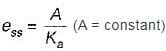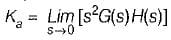= Finite for type-2 system
So, ess = Some finite value

Test: Time Domain Analysis of Control Systems- 3 - Question 5

The open loop transfer function of a unity-feedback control system with unit step input is given by: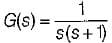The settling time of the system for 5% tolerance band is,

Detailed Solution for Test: Time Domain Analysis of Control Systems- 3 - Question 5

Characteristic equation is: 1 + G(s) H(s) = 0 or, s2 + s + 1 = 0
Here,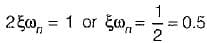For 5% tolerance band, settling time is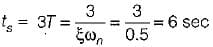Test: Time Domain Analysis of Control Systems- 3 - Question 6

The integral of a ramp function becomes

Test: Time Domain Analysis of Control Systems- 3 - Question 7

Consider a unity feedback control system as shown in figure below: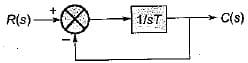The error signal e(t) for a unit ramp input for T ≥ 0 is

Detailed Solution for Test: Time Domain Analysis of Control Systems- 3 - Question 7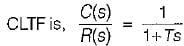Given,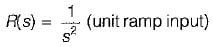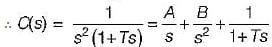Here, A = -T, 6 = 1, C = T2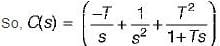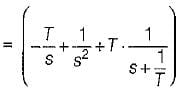Taking inverse Laplace transform on both sides, we get:
C(t) = (-T + t + Te-t/T)
∴ Error signal,
e(t) = c(t) - r(t)
= c(t) - t = - T + Te-t/T
= T(1 - e-t/T)

Test: Time Domain Analysis of Control Systems- 3 - Question 8

A second order system has a closed loop transfer function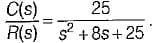If the system is initially at rest and subjected to a unit step input at t = 0, the third peak in the response will occur at

Detailed Solution for Test: Time Domain Analysis of Control Systems- 3 - Question 8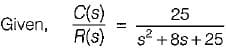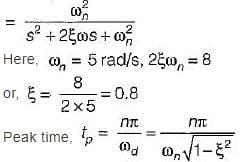For 3rd peak, n = 5
(3rd overshoot is the third peak)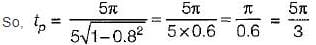Test: Time Domain Analysis of Control Systems- 3 - Question 9

A closed servo system is represented by the differential equation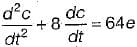where c is the displacement of the output shaft r is the displacement of the input shaft anc e = r - c.
The damping ratio and natural frequency for this system are respectively

Detailed Solution for Test: Time Domain Analysis of Control Systems- 3 - Question 9

Given,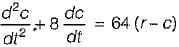or, s2C(s) + 8s C(s) = 64 [R(s) - C(s)]
or,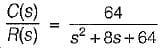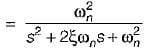and 2ξωn = 8 or ξ = 0.5

Test: Time Domain Analysis of Control Systems- 3 - Question 10

The poles of a closed loop control system art located at -1 ± j.
For the given system match List-I (Time domain specifications) with List - II (Values) and select the correct answer using the codes given below the lists
List - I
A. Damping ratio
C. Damping factor
D. Settling time (in secs)
List - II
1. 4.0
2. 1.0
3. 0.707
4. 1.414
Codes: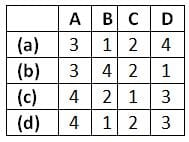Detailed Solution for Test: Time Domain Analysis of Control Systems- 3 - Question 10

Given, poles are at s = -1 ± j ∴ Characteristic equation is
(s+1)2 + 1 =0
or, s2 + 2s + 2 = 0
Comparing above equation with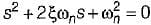we have, ωn = √2 rad/sec
and 2ξωn = 2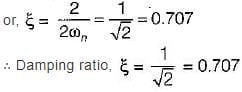Undamped natural frequency,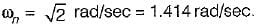Damping factor = ξωn = 1
Settling time (For 2% tolerance band) is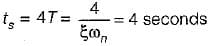Damped natural frequency is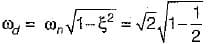Test: Time Domain Analysis of Control Systems- 3 - Question 11

Consider the block diagram of a control system shown below.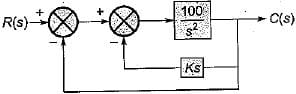What is the value of gain k such that the system is non oscillatory yet has the lowest possible settling time to step input?

Detailed Solution for Test: Time Domain Analysis of Control Systems- 3 - Question 11

Forward gain,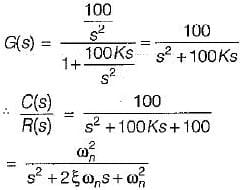Here, ωn = 10 rad/s and 2ξωn > = 100 K
or,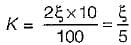For no-oscillation and to have lowest possible settling time ξ = 1.
∴ K = 1/5 = 0.2

Test: Time Domain Analysis of Control Systems- 3 - Question 12

Assertion (A): The "dominant pole" concept is used to control the dynamic performance of the system, whereas the “insignificant poles” are used for the purpose of. ensuring that the controller transfer function can be realized by physical components.
Reason (R): if the magnitude of the real part of a pole is at least 5 to 10 times that of a dominant pole or a pair of complex dominant poles, the pole may be regarded as insignificant in so far as the transient response is concerned.

Detailed Solution for Test: Time Domain Analysis of Control Systems- 3 - Question 12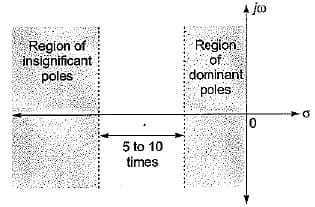Both assertion and reason are true. However, the correct explanation of assertion is that the poles having real part magnitude 5 to 10 times of the magnitude of real part of dominant poles doesn't affect the transient response (dynamic performance) of the system to a great extent due to which they are termed as insignificant poies and hence are neglected for transient stability study purpose.

Test: Time Domain Analysis of Control Systems- 3 - Question 13

Assertion (A): The step-function input represents an instantaneous change in the reference input.
Reason (R): If the input is an angular position of a mechanical shaft, a step input represents the sudden rotation of the shaft.
(a) Both A and R are true and R is a correct explanation of A.
(b) Both A and R are true but R is not a correct explanation of A.
(c) A is true but R is false.
(d) A is false but R is true.

Test: Time Domain Analysis of Control Systems- 3 - Question 14

Consider the following statements related to second order control system with unit step input:
1. The right-half s-plane corresponds to negative damping.
2. Negative damping gives a response that doesn’t grows in magnitude without bound with time.
3. The imaginary axis corresponds to zero damping.
4. The maximum overshoot is often used to measure the relative stability of a control system.
5. Higher the damping in the system larger is the peak overshoot in the transient response of the system.
Which of the above statements is/are correct?

Detailed Solution for Test: Time Domain Analysis of Control Systems- 3 - Question 14

Negative damping gives a response that grows in magnitude without bound in time. Hence, statement-2 is false.
Since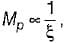therefore higher is the damping in the system, less is the peak overshoot of the system. Hence, statement-5 is not correct

Test: Time Domain Analysis of Control Systems- 3 - Question 15

Which of the following is not true regarding addition of a pole to the forward-path transfer function?

Detailed Solution for Test: Time Domain Analysis of Control Systems- 3 - Question 15

With addition of a pole to the forward-path transfer function, bandwidth will reduce.
Since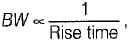therefore rise time will increase.
Since rise time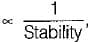therefore stability will decrease.

Test: Time Domain Analysis of Control Systems- 3 - Question 16

For a second-order prototype system, when the undamped natural frequency is increased, the maximum overshoot of the output will

Detailed Solution for Test: Time Domain Analysis of Control Systems- 3 - Question 16

We know that, maximum overshot is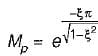Mp is independent of wn. So M∝ ξ only.

Test: Time Domain Analysis of Control Systems- 3 - Question 17

Consider a unity feedback system shown in figure below where, K > 0 and a > 0.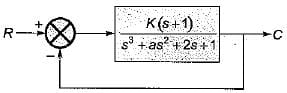What are the values of k and a respectively so that the system oscillates at a frequency of 2 rad/sec?

Detailed Solution for Test: Time Domain Analysis of Control Systems- 3 - Question 17

Given,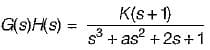Now, 1 + G(s)H(s) = 0
or, s3 + as2 + (2 + K) s + (1 + K) = 0
Routh’s array is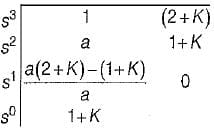For oscillation, we have: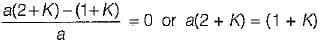Also, oscillating frequency will be given by roots of A2(s) = 0.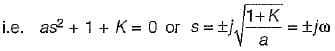Given,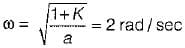We see that only option (d) is matching, i.e. for K = 2 and a = 0.75,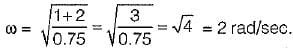Test: Time Domain Analysis of Control Systems- 3 - Question 18

The transient response of a system is mainly due to

Test: Time Domain Analysis of Control Systems- 3 - Question 19

A second order system has a damping ratio ξ and undamped natural frequency of oscillation ωn respectively. The settling time at 5% of tolerance band of the system is

Detailed Solution for Test: Time Domain Analysis of Control Systems- 3 - Question 19

For 2% tolerance band,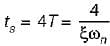For 5% tolerance band,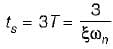Test: Time Domain Analysis of Control Systems- 3 - Question 20

The unit impulse response of a linear time invariant second order system is
g(t) = 10e-8t sin 6t (t ≥ C)
The natural frequency and the damping ratio of the system are respectively

Detailed Solution for Test: Time Domain Analysis of Control Systems- 3 - Question 20

Given, c(t) = g(t) = 10e-8t sin6t
Here,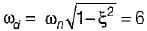and ξωn = 8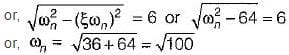So,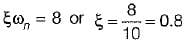## GATE Electrical Engineering (EE) 2024 Mock Test Series

23 docs|285 tests
Information about Test: Time Domain Analysis of Control Systems- 3 Page
In this test you can find the Exam questions for Test: Time Domain Analysis of Control Systems- 3 solved & explained in the simplest way possible. Besides giving Questions and answers for Test: Time Domain Analysis of Control Systems- 3, EduRev gives you an ample number of Online tests for practice

## GATE Electrical Engineering (EE) 2024 Mock Test Series

23 docs|285 tests